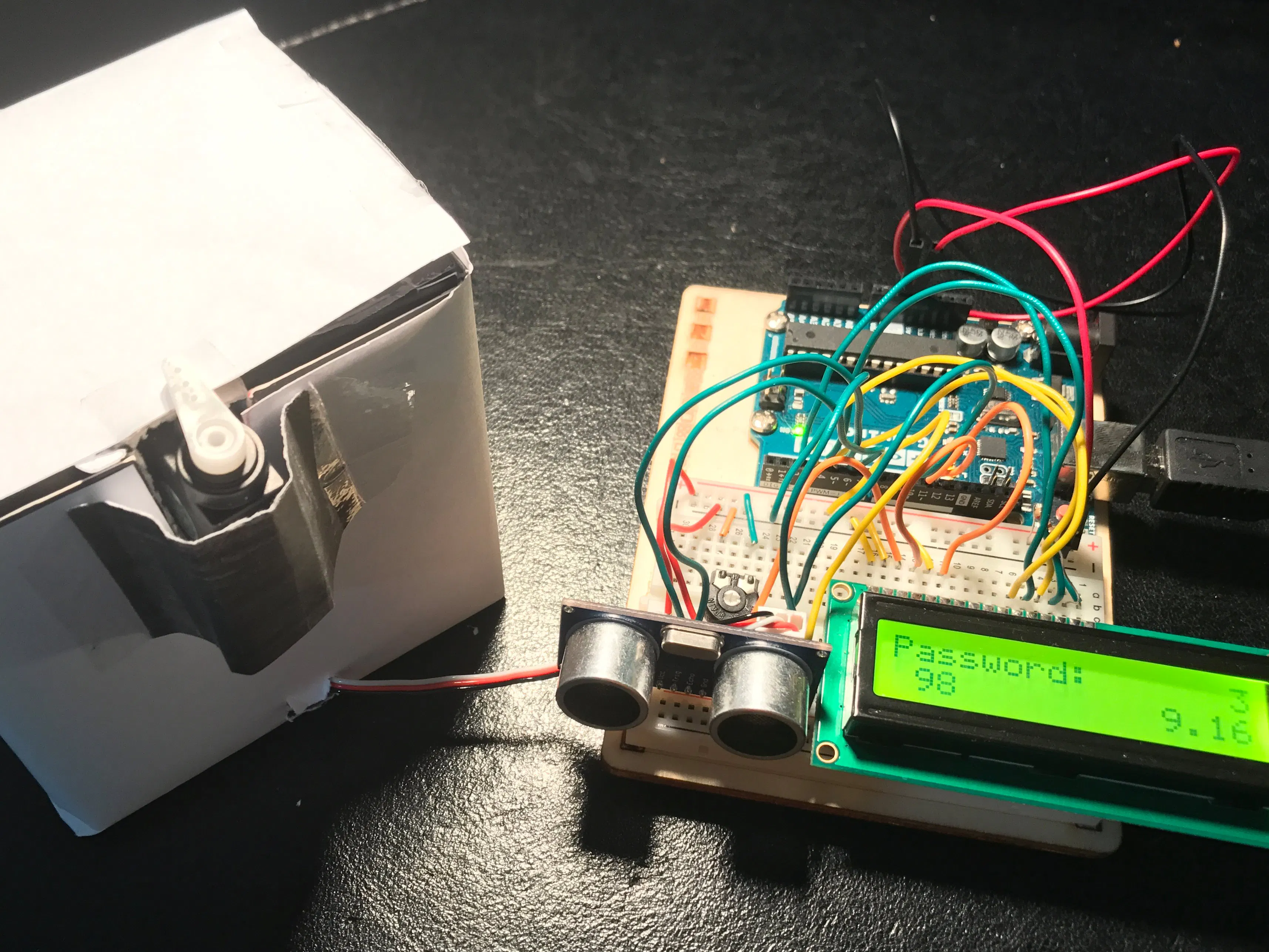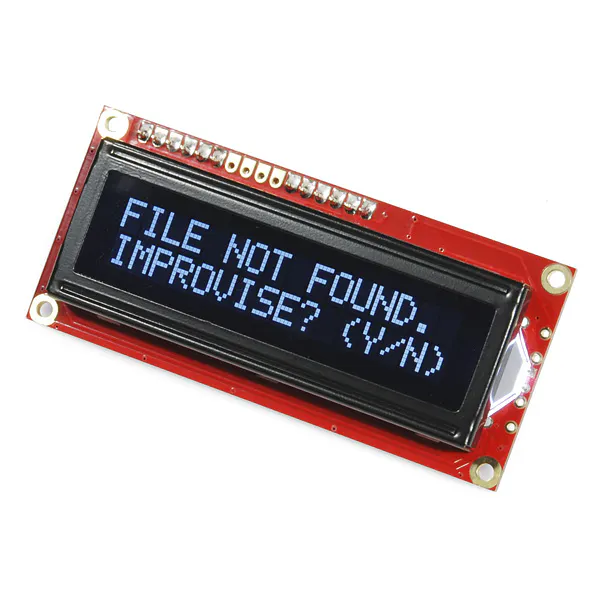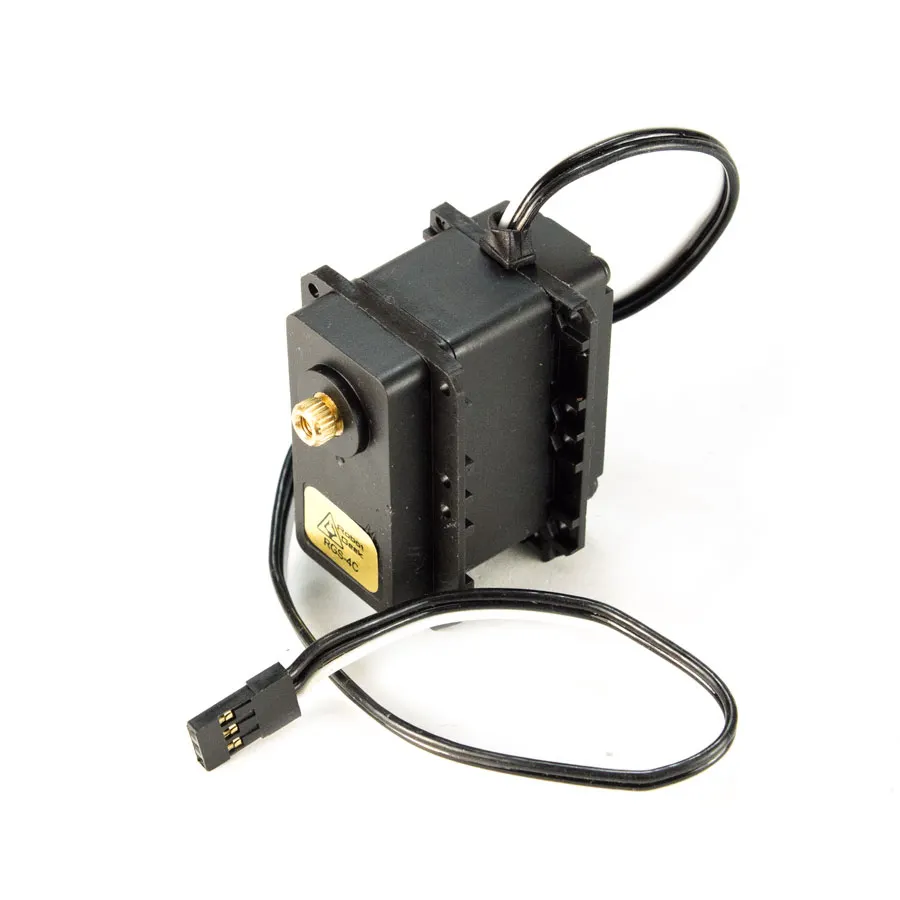# Ultrasonic Lock

Anything can be used as a key as long as you know the password.

BeginnerProtip1 hour6,226## Things used in this project

### Hardware componentsSparkFun Serial Enabled 16x2 LCD - White on Black 3.3V
×1RobotGeek Continuous Rotation Servo
×1Ultrasonic Sensor - HC-SR04 (Generic)
×1×1Arduino UNO & Genuino UNO
×1

### Software apps and online servicesArduino IDE

## Code

### Ultrasonic Lock-Code

C/C++
```#include<Servo.h>
#include<LiquidCrystal.h>

LiquidCrystal lcd(12,11,5,4,3,2);
Servo myServo;

const int trigPin = 6;
const int echoPin = 7;
const int sound = 340;

float duration;
float distance;
float distanceLoop;
int checkPass;//array with 4 values(initial every elemnet is equal with 0)
int number;
int count = 0;
int sizeArray;
float distanceCheck;// to check when it is ready to close box

void setup(){
Serial.begin(9600);
lcd.begin(16,2);
myServo.attach(9);
pinMode(trigPin,OUTPUT);
pinMode(echoPin,INPUT);
sizeArray = sizeof(pass)/2;//size of array
int checkPass;
myServo.write(120);
delay(1000);
}

void loop(){
lcd.clear();
lcd.setCursor(0,0);
lcd.setCursor(1,0);
for(int num = 0; num <= sizeArray ; num ++){//takes enough measurements(password keys) as the number of elements of password
for(int i = 3; i > 1 ;i--){//countdown before a measurement(password key) is taken
lcd.setCursor(15,0);
lcd.print(i);
lcd.setCursor(12,2);
lcd.print(Distance());
delay(1000);
}
distanceLoop = Distance();//measurement is taken
number = check(distanceLoop,pass[num]);//rounds up number
checkPass[num] = number;//assigns measurement to the array
lcd.setCursor(num + 1,2);
lcd.print(number);
}
for(int i = 0; i < 4 ; i ++){
Serial.println(checkPass[i]);
}
count = checkCount(0,checkPass,pass,3);/*compares 2 arrays to check if the password is correct
for every wrong value count variable increase by one*/
if(count != 0){
lcd.clear();
myServo.write(120);
delay(5000);
}
else{
lcd.clear();
myServo.write(30);
distanceCheck = Distance();
while(distanceCheck > 5){
distanceCheck = Distance();
myServo.write(30);
delay(500);
}
myServo.write(120);
delay(1000);
}
}

float Distance(){//measures distance in cm
digitalWrite(trigPin,LOW);
delayMicroseconds(15);
digitalWrite(trigPin,HIGH);
delayMicroseconds(2000);
digitalWrite(trigPin,LOW);
delayMicroseconds(10);
duration = pulseIn(echoPin,HIGH);
distance = sound * duration/20000;
return distance;
}

int check(float msr,float nUm){//rounds up number
if(msr >= nUm - 0.5 && msr <= nUm + 0.5){
msr = nUm;
return msr;
}
else{
return (int) msr;

}
}

int checkCount(int cnt,int checkPass[],int pass[],int times){//check password
for(int num = 0; num <= times ; num ++){
if(checkPass[num] == pass[num]){
cnt = cnt;
}
else{
cnt += 1;
}
}
return cnt;
}
```

## Credits

### IoannisD

0 projects • 1 follower